# 泊松白噪声驱动的一维随机波动方程One-Dimensional Stochastic Wave Equations Driven by Poisson White Noise

DOI: 10.12677/PM.2021.114068, PDF, HTML, XML, 下载: 20  浏览: 64  科研立项经费支持

Abstract: Partial differential equation is a kind of deterministic equations, although with wide applications in many fields, but not a good description of uncertain situation, thus to explore the partial differential equation of random noise drive equation is very important, so this paper mainly use the Picard iteration and Gronwall inequality is proved under certain conditions poisson white noise driven one-dimensional random wave equation of mild existence and uniqueness of solution.

1. 引言

$\left\{\begin{array}{l}\frac{{\partial }^{2}u}{\partial {t}^{2}}={a}^{2}\frac{{\partial }^{2}u}{\partial {x}^{2}}\\ u\left(0,x\right)=\phi \left(x\right)\\ {u}_{t}\left(0,x\right)=\psi \left( x \right)\end{array}$

$\left\{\begin{array}{l}\frac{{\partial }^{2}u}{\partial {t}^{2}}\left(t,x,\omega \right)=\frac{{\partial }^{2}u}{\partial {x}^{2}}\left(t,x,\omega \right)+f\left(t,u\left(t,x\right)\right)+{\int }_{u}g\left(t,x,u\left(t,x,\omega \right);y\right){\eta }_{t}\left(\text{d}y,\omega \right)\\ u\left(0,x\right)=0\\ {u}_{t}\left(0,x\right)=0\end{array}$ (1)

$\begin{array}{c}u\left(t,x,\omega \right)={\int }_{0}^{t+}{\int }_{u}{\int }_{R}\left({G}_{t-s}\left(x-z\right)g\left(s,z,u\left(s,-z,\omega \right);y\right)\right)\text{d}z\text{ }q\left(\text{d}s,\text{d}y,\omega \right)\\ \text{\hspace{0.17em}}+{\int }_{0}^{t}{\int }_{R}\left({G}_{t-s}\left(x-z\right)f\left(s,u\left(s,z\right)\right)\right)\text{d}s\text{d}z\end{array}$ (2)

2. 主要内容

2.1. 假设

2.1.1. 假设1

1) $u\left(t,x,\omega \right)$$\left\{{F}_{t}\right\}$ -适应过程；

2) $u\left(t,x,\omega \right)$${L}^{2}\left(\Omega \right)$ 值的随机过程，关于变量 $t\in \left[0,\infty \right)$ 是右连左极限存在的；

3) 固定的t，x，方程成立。

2.1.2. 假设2

(c1)： ${\int }_{U}{\left[g\left(t,x,z;y\right)\right]}^{2}v\left(\text{d}y\right)\le {K}_{T}\left(x\right)|z|$

(c2)： ${|f\left(t,z\right)|}^{2}\le a\left(t\right)+k\left(t\right)|z|$

(c3)： ${\int }_{U}{\left[g\left(t,x,{z}_{1};y\right)-g\left(t,x,{z}_{2};y\right)\right]}^{2}v\left(\text{d}y\right)\le {K}_{T}\left(x\right){|{z}_{1}-{z}_{2}|}^{2}$

(c4)： ${|f\left(t,{z}_{1}\right)-f\left(t,{z}_{2}\right)|}^{2}\le {B}_{T}|{z}_{1}-{z}_{2}|$

2.2. 推导过程

$u\left(t,x,\omega \right)={I}_{1}+{I}_{2}$，其中：

${I}_{1}:{\int }_{0}^{t+}{\int }_{u}{\int }_{R}\left({G}_{t-s}\left(x-z\right)g\left(s,z,u\left(s,-z,\omega \right);y\right)\right)\text{d}z\text{ }q\left(\text{d}s,\text{d}y,\omega \right)$

${I}_{2}:{\int }_{0}^{t}{\int }_{R}{G}_{t-s}\left(x-z\right)f\left(s,u\left(s,z\right)\right)\text{d}s\text{d}z$

${I}_{1}:{u}_{n+1}\left(t,x,\omega \right)={\int }_{0}^{t+}{\int }_{u}{\int }_{R}\left({G}_{t-s}\left(x-z\right)g\left(s,z,u\left(s,-z,\omega \right);y\right)\right)\text{d}z\text{ }q\left(\text{d}s,\text{d}y,\omega \right)={I}_{1}\left(t,x,\omega \right)$ (3)

${I}_{2}:{u}_{n+1}\left(t,x\right)={\int }_{0}^{t}{\int }_{R}\left({G}_{t-s}\left(x-z\right)f\left(s,{u}_{n}\left(s,z\right)\right)\right)\text{d}z\text{d}s={I}_{1}\left(t,x\right)$ (4)

${I}_{1}:$

${h}_{t,x}\left(s,y,\omega \right):={\int }_{R}\left({G}_{t-s}\left(x-z\right)g\left(s,z,{u}_{n}\left(s,z,\omega \right);y\right)\right)\text{d}z\in H$

${\mu }_{n}^{\left(m\right)}\left(s,z,\omega \right):={\mu }_{n}\left(0,z,\omega \right)+\underset{k=0}{\overset{{2}^{m}-1}{\sum }}\text{ }\text{ }{\mu }_{n}\left(\frac{kt}{{2}^{m}},z,\omega \right){1}_{\left(\frac{kt}{{2}^{m}},\frac{\left(k+1\right)t}{{2}^{m}}\right)}\left(s\right),m\in N$

${\mu }_{n}^{\left(m\right)}\left(s,z,\omega \right)$$\left\{{F}_{s}\right\}$ -可料的。

${h}_{t,x}^{m}\left(s,y,\omega \right):={\int }_{R}{G}_{t-s}\left(x-z\right)g\left(s,z,{u}_{n}^{\left(m\right)}\left(s,z,\omega \right);y\right)\text{d}z,m\in N.$

1) 证明 ${h}_{t,x}^{m}\left(s,y,\omega \right)$$\left\{{F}_{s}\right\}$ -可料的。

2) 证明 ${h}_{t,x}^{m}\left(s,y,\omega \right)$$B\left[0,T\right]\otimes R\otimes F/B\left(R\right)$ -可测的，由Fubini定理，只需证明：

${\int }_{R}{G}_{t-s}\left(x-z\right)|g\left(s,z,{u}_{n}^{\left(m\right)}\left(s,z,\omega \right);y\right)|\text{d}z<\infty ,$ (5)

(可以由下面的证明得到)。

3) $E{\int }_{0}^{t}{\int }_{u}{\left[{h}_{t,x}^{m}\left(s,y,\omega \right)\right]}^{2}v\left(\text{d}y\right)\text{d}s<\infty$

$\begin{array}{l}{\int }_{R}{G}_{t-s}\left(x-z\right)|g\left(s,z,{u}_{n}^{\left(m\right)}\left(s,z,\omega \right);y\right)|\text{d}z\\ \le {\left\{{\int }_{R}{G}_{t-s}\left(x-z\right)\text{d}z\right\}}^{\frac{1}{2}}{\left\{{\int }_{R}{{G}_{t-s}\left(x-z\right)\left(g\left(s,z,\omega \right);y\right)\right]}^{2}\text{d}z\right\}}^{\frac{1}{2}}<\infty \end{array}$

$\begin{array}{l}{\int }_{u}{\int }_{R}{G}_{t-s}\left(x-z\right){\left[g\left(s,z,{U}_{n}^{\left(m\right)}\left(s,z,\omega \right);y\right)\right]}^{2}\text{d}zv\left(\text{d}y\right)\\ \le T{\int }_{R}{\int }_{u}{G}_{t-s}\left(x-z\right){\left[g\left(s,z,{U}_{n}^{\left(m\right)}\left(s,z,\omega \right);y\right)\right]}^{2}\text{d}zv\left(\text{d}y\right)\\ =T{\int }_{R}{G}_{t-s}\left(x-z\right){\left[g\left(s,z,{U}_{n}^{\left(m\right)}\left(s,z,\omega \right);y\right)\right]}^{2}\text{d}zv\left(\text{d}y\right)\\ \le T{\int }_{R}{G}_{t-s}\left(x-z\right){K}_{T}\left(z\right)|{U}_{n}^{\left(m\right)}\left(s,z,\omega \right)|\text{d}z\\ \le T{\left\{{\int }_{R}{G}_{t-s}\left(x-z\right){K}_{T}{\left(z\right)}^{2}\text{d}z\right\}}^{\frac{1}{2}}{\left\{2{\int }_{R}{G}_{t-s}\left(x-z\right){\left(|{U}_{n}^{\left(m\right)}\left(s,z,\omega \right)|\right)}^{2}\text{d}z\right\}}^{\frac{1}{2}}\\ \le \sqrt{2}T{\left\{{\int }_{R}{K}_{T}{\left(Z\right)}^{2}\text{d}z\right\}}^{\frac{1}{2}}{\left\{{\int }_{R}{\left(|{U}_{N}^{\left(m\right)}\left(s,z,\omega \right)|\right)}^{2}\text{d}z\right\}}^{\frac{1}{2}}<\infty \end{array}$ (6)

$E\left\{{\int }_{0}^{T}{\int }_{U}{\left[{h}_{t,x}^{m}\left(s,y,\omega \right)\right]}^{2}v\left(\text{d}y\right)\text{d}s\right\}\le TE\left\{{\int }_{0}^{T}{\int }_{U}{\int }_{R}{G}_{t-s}\left(x-z\right){\left[g\left(s,z,{u}_{n}^{\left(m\right)}\left(s,z,\omega \right);y\right)\right]}^{2}\text{d}zv\left(\text{d}y\right)\text{d}s\right\}<\infty$

(通过文献  可得引理2的证明过程，在此省略)

(这部分与文献  中证明类似，但为了保持文章完整性，在此列出具体过程)

$\begin{array}{l}E{\left[{J}_{t}\left(r,{x}_{1},.\right)-{J}_{t}\left(r,{x}_{2},.\right)\right]}^{2}\\ =E\left\{{{\int }_{0}^{r+}\left[{\int }_{R}|{G}_{t-s}\left({x}_{1}-z\right)-{G}_{t-s}\left({x}_{2}-z\right)|g\left(s,z,{u}_{n}\left(s,z\right);y\right)\text{d}z\right]}^{2}v\left(\text{d}y\right)\text{d}s\right\}\\ \le E\left\{{\int }_{0}^{r+}{\int }_{R}|{G}_{t-s}\left({x}_{1}-z\right)-{G}_{t-s}\left({x}_{2}-z\right)|\text{d}z×{\int }_{R}|{G}_{t-s}\left({x}_{1}-z\right)-{G}_{t-s}\left({x}_{2}-z\right)|{\int }_{u}{\left\{g\left(s,z,{u}_{n}\left(s,z,.\right);y\right)\right\}}^{2}\right\}v\left(\text{d}y\right)\text{d}z\text{d}s\\ \le E\left\{{\int }_{0}^{r+}{\int }_{R}|{G}_{t-s}\left({x}_{1}-z\right)-{G}_{t-s}\left({x}_{2}-z\right)|\text{d}z×{\int }_{R}\left\{|{G}_{t-s}\left({x}_{1}-z\right)-{G}_{t-s}\left({x}_{2}-z\right)|{K}_{T}\left(Z\right)|{u}_{n}\left(s,z,.\right)|\text{d}z\text{d}s\right\}\right\}\end{array}$

$\begin{array}{l}{\int }_{R}|{G}_{t-s}\left({x}_{1}-z\right)-{G}_{t-s}\left({x}_{2}-z\right)|\text{d}z\\ ={\int }_{R}|{1}_{\left\{{x}_{1}-\left(t-s\right)\le z\le {x}_{1}+\left(t-s\right)\right\}}-{1}_{\left\{{x}_{2}-\left(t-s\right)\le z\le {x}_{2}+\left(t-s\right)\right\}}|\text{d}z\\ =4\left(t-s\right){1}_{\left\{s\ge t-\frac{\left({x}_{2}-{x}_{1}\right)}{2}\right\}}+2\left({x}_{2}-{x}_{1}\right){1}_{\left\{s

${\int }_{R}|{G}_{t-s}\left({x}_{1}-z\right)-{G}_{t-s}\left({x}_{2}-z\right)|\text{d}z=4\left(t-s\right){1}_{\left\{s\ge t-\frac{\left({x}_{2}-{x}_{1}\right)}{2}\right\}}+2\left({x}_{2}-{x}_{1}\right){1}_{\left\{s

$\begin{array}{l}E{\left[{I}_{1}\left(t,{x}_{1},.\right)-{I}_{1}\left(t,{x}_{2},.\right)\right]}^{2}\\ =E{\left[{J}_{t}\left(t,{x}_{1},.\right)-{J}_{t}\left(t,{x}_{2},.\right)\right]}^{2}\\ \le E\left\{{\int }_{0}^{t}{\int }_{R}|{G}_{t-s}\left({x}_{1}-z\right)-{G}_{t-s}\left({x}_{2}-z\right)|\text{d}z\right\}×\left[{\int }_{R}|{G}_{t-s}\left({x}_{1}-z\right)-{G}_{t-s}\left({x}_{2}-z\right)|{K}_{T}\left(Z\right)|{u}_{n}\left(s,z,.\right)|\text{d}z\right]\text{d}s\\ \le E\left\{{\int }_{0}^{t}{\int }_{R}|{G}_{t-s}\left({x}_{1}-z\right)-{G}_{t-s}\left({x}_{2}-z\right)|\text{d}z×{\left[{\int }_{R}{|{G}_{t-s}\left({x}_{1}-z\right)-{G}_{t-s}\left({x}_{2}-z\right)|}^{\frac{4+2\epsilon }{\epsilon }}\text{d}z\right]}^{\frac{4+2\epsilon }{\epsilon }}\\ \text{\hspace{0.17em}}\text{\hspace{0.17em}}{\left[{\int }_{R}{\left({K}_{T}\left(Z\right)\right)}^{2+\epsilon }\text{d}z\right]}^{\frac{1}{2+\epsilon }}×{\left[{\int }_{R}{\left\{{u}_{n}\left(s,z,.\right)\right]}^{2}\text{d}z\right]}^{\frac{1}{2}}\right\}\text{d}s\end{array}$

$c\left(s\right)={\left\{{\int }_{R}{\left({K}_{T}\left(z\right)\right)}^{2+\epsilon }\text{d}z\right\}}^{\frac{1}{2+\epsilon }}E{\left[{\int }_{R}{\left[{u}_{n}\left(s,z,\cdot \right)\right]}^{2}\text{d}z\right]}^{\frac{1}{2}}$

${\mathrm{sup}}_{s\in \left\{0,T\right]}C\left(s\right)<\infty$

$\begin{array}{l}E{\left[{I}_{1}\left(t,{x}_{1},\cdot \right)-{I}_{1}\left(t,{x}_{2},\cdot \right)\right]}^{2}\\ \le {\mathrm{sup}}_{s\in \left\{0,T\right]}C\left(s\right)\left[{2}^{h\left(\epsilon \right)-1}\frac{1}{h\left(\epsilon \right)+1}{|{x}_{2}-{x}_{1}|}^{h\left(\epsilon \right)+1}+{2}^{h\left(\epsilon \right)}{|{x}_{2}-{x}_{1}|}^{h\left(\epsilon \right)}t-{2}^{h\left(\epsilon \right)-1}{|{x}_{2}-{x}_{1}|}^{h\left(\epsilon \right)+1}\right]\\ \le {\mathrm{sup}}_{s\in \left\{0,T\right]}C\left(s\right){\int }_{0}^{t}{\left[{\int }_{R}|{G}_{t-s}\left({x}_{1}-z\right)-{G}_{t-s}\left({x}_{2}-z\right)|\text{d}z\right]}^{h\left(\epsilon \right)}\text{d}s\\ \le {\mathrm{sup}}_{s\in \left\{0,T\right]}C\left(s\right)\left[{\int }_{t-\frac{|{x}_{2}-{x}_{1}|}{2}}^{t}{4}^{h\left(\epsilon \right)}{\left(t-s\right)}^{h\left(\epsilon \right)}\text{d}s+{\int }_{0}^{t-\frac{|{x}_{2}-{x}_{1}|}{2}}{2}^{h\left(\epsilon \right)}{\left({x}_{2}-{x}_{1}\right)}^{h\left(\epsilon \right)}\text{d}s\right]\\ \le {\mathrm{sup}}_{s\in \left\{0,T\right]}C\left(s\right)\left[{2}^{h\left(\epsilon \right)-1}\frac{1}{h\left(\epsilon \right)+1}{|{x}_{2}-{x}_{1}|}^{h\left(\epsilon \right)+1}+{2}^{h\left(\epsilon \right)}{|{x}_{2}-{x}_{1}|}^{h\left(\epsilon \right)}t-{2}^{h\left(\epsilon \right)-1}{|{x}_{2}-{x}_{1}|}^{h\left(\epsilon \right)+1}\right]\end{array}$

${C}_{T}=\mathrm{max}\left\{{\mathrm{sup}}_{s\in \left\{0,T\right]}c\left(s\right),{2}^{h\left(\epsilon \right)-1}\frac{1}{h\left(\epsilon \right)+1},{2}^{h\left(\epsilon \right)}T\right\}$

$\mathrm{sup}E{\left[{I}_{1}\left(t,{x}_{1},\cdot \right)-{I}_{1}\left(t,{x}_{2},\cdot \right)\right]}^{2}\le {C}_{T}{|{x}_{2}-{x}_{1}|}^{h\left(\epsilon \right)}t\in \left\{0,T\right].$

${I}_{1}:$

$\begin{array}{l}E{\int }_{R}{\left[{U}_{n+1}\left(t,x,.\right)\right]}^{2}\text{d}x\\ =E{\int }_{R}{\left[{I}_{1}\left(t,{x}_{1},\cdot \right)\right]}^{2}\text{d}s={E}_{K}{\left\{{\int }_{t}\left(t,s,.\right)\right\}}^{2}\text{d}x\\ ={\int }_{R}E{\left[{\int }_{0}^{t}{\int }_{R}{G}_{t-s}\left(x-z\right)g\left(s,z,{u}_{z}\left(s,z,\omega \right);y\right)\right]}^{2}\text{d}zv\left(\text{d}y\right)\text{d}s\right\}\text{d}x\\ \le T{\int }_{R}E\left\{{\int }_{0}^{t}{\int }_{u}{\int }_{R}{G}_{t-s}\left(x-z\right){\left\{g\left(s,z,{u}_{z}\left(s,z,\omega \right);y\right)\right\}}^{2}\right\}\\ \le T{\int }_{R}E\left\{{\int }_{0}^{t}{\int }_{R}\left[{\int }_{R}{G}_{t-s}\left(x-z\right)\text{d}z\right]{K}_{T}\left(z\right)|{u}_{n}\left(s,z,\cdot \right)|\text{d}z\text{d}s\end{array}$

$\begin{array}{l}\le {T}^{2}E\left\{{\int }_{0}^{t}{\int }_{R}{K}_{T}\left(z\right)|{u}_{n}\left(s,z,\cdot \right)|\text{d}z\text{d}s\right\}\\ \le {T}^{2}E\left\{{\int }_{0}^{t}{\left\{{\int }_{R}{\left[{K}_{T}\left(Z\right)\right]}^{2}\text{d}z\right\}}^{\frac{1}{2}}{\int }_{0}^{t}{\left\{{\int }_{R}{|{u}_{n}\left(s,z,\cdot \right)|}^{2}\text{d}z\text{d}s\right\}}^{\frac{1}{2}}\\ \le {T}^{2}{\int }_{0}^{t}{\left\{{\int }_{R}{\left[{K}_{T}\left(z\right)\right]}^{2}\text{d}z\right\}}^{\frac{1}{2}}E{\left[{\int }_{R}{|{u}_{n}\left(s,z,\cdot \right)|}^{2}\text{d}z\right]}^{\frac{1}{2}}\text{d}s<\infty \end{array}$

${I}_{2}:$

${\int }_{R}|{G}_{t-s}\left(x-z\right)f\left(s,{u}_{n}\left(s,z\right)\right)|\text{d}z\le {\left\{{\int }_{R}{G}_{t-s}\left(x-z\right)\text{d}z\right\}}^{\frac{1}{2}}{\left\{{\int }_{R}{G}_{t-s}\left(x-z\right){\left[{\int }^{\text{​}}\left(s,{u}_{n}\left(s,z\right)\right)\right]}^{2}\text{d}z\right\}}^{\frac{1}{2}}$

$\begin{array}{l}{\left\{{\int }_{R}{G}_{t-s}\left(x-z\right){\left[f\left(s,{u}_{n}\left(s,z\right)\right)\right]}^{2}\text{d}z\right\}}^{\frac{1}{2}}\\ \le 2\left({\int }_{R}\left(a\left(s\right)+k\left(s\right)|{u}_{n}\left(s,z\right)|\right)\text{d}z+1\right)\\ \le {‖a‖}_{1}+{‖k‖}_{2}{‖{U}_{n}\left(s\right)‖}_{2}+1<\infty \end{array}$

2.3. 存在性证明

${I}_{1}:$

${F}_{n}\left(t\right):=E\left\{{\int }_{R}{\left[{u}_{n+1}\left(s,z,\cdot \right)-{u}_{n}\left(s,z,\cdot \right)\right]}^{2}\text{d}x\right\}$ 其中 $t\in \left[0,T\right]$$n\in N$，由公式(3)和假设(c3)，我们可以得到：

$\begin{array}{l}E{\int }_{R}{\left\{{\int }_{0}^{t+}{\int }_{U}{\int }_{R}{G}_{t-s}\left(x-z\right)×\left[g\left(s,z,{u}_{n}\left(s,z,\omega \right);y\right)-g\left(s,z,{u}_{n-1}\left(s,z,\omega \right);y\right)\right]\text{d}zq\left(\text{d}s,\text{d}y,\cdot \right)\right\}}^{2}\text{d}x\\ =E{{\int }_{R}{\int }_{0}^{t}{\int }_{U}\left[{\int }_{R}{G}_{t-s}\left(x-z\right)×\left[g\left(s,z,{u}_{n}\left(s,z,\omega \right);y\right)-g\left(s,z,{u}_{n-1}\left(s,z,\omega \right);y\right)\right]\text{d}z\right]}^{2}v\left(\text{d}y\right)\text{d}s\text{d}x\\ \le {L}_{T}E{\int }_{R}{\int }_{0}^{t}{\int }_{R}{G}_{t-s}\left(x-z\right){\left[{u}_{n}\left(s,z,\omega \right)-{u}_{n-1}\left(s,z,\omega \right)\right]}^{2}\text{d}z\text{d}s\text{d}x\\ ={L}_{T}E{\int }_{R}{\int }_{0}^{t}\left\{{\int }_{R}{G}_{t-s}\left(x-z\right)\text{d}x\right\}{\left[{u}_{n}\left(s,z,\omega \right)-{u}_{n-1}\left(s,z,\omega \right)\right]}^{2}\text{d}z\text{d}s\\ \le T{L}_{T}{\int }_{0}^{t}E{\int }_{R}{\left[{u}_{n}\left(s,z,\omega \right)-{u}_{n-1}\left(s,z,\omega \right)\right]}^{2}\text{d}z\text{d}s\\ ={C}_{T}{L}_{T}{\int }_{0}^{t}{F}_{n-1}\left(s\right)\text{d}s\end{array}$

${F}_{n}\left(t\right)\le {\left[{G}_{T}{L}_{T}\right]}^{n-1}{\int }_{0}^{t}\text{d}{t}_{1}{\int }_{0}^{{t}_{1}}\text{d}{t}_{2}\cdots {\int }_{0}^{{t}_{n-2}}{F}_{1}\left({t}_{n-1}\right)\text{d}{t}_{n-1}$

$\begin{array}{c}{F}_{1}\left(t\right)=E\left\{{\int }_{R}{\left[{u}_{2}\left(t,x,\cdot \right)-{u}_{1}\left(t,x,\cdot \right)\right]}^{2}\text{d}x\right\}\\ \le {C}_{T}{\int }_{R}{\int }_{0}^{t}{\int }_{R}{G}_{t-s}\left(x-z\right){K}_{T}\left(z\right)|{u}_{1}\left(s,z,\cdot \right)|\text{d}z\text{d}s\\ ={C}_{T}{\int }_{R}{\int }_{0}^{t}\left\{{\int }_{R}{G}_{t-s}\left(x-z\right)\text{d}x\right\}{K}_{T}\left(z\right)\text{d}z\text{d}s\\ \le T{C}_{T}{\int }_{0}^{t}{\int }_{R}{K}_{T}\left(z\right)\text{d}z\text{d}s\end{array}$

$\begin{array}{l}E{\int }_{R}{\left\{{\int }_{0}^{t+}{\int }_{R}{G}_{t-s}\left(x-z\right)×\left\{f\left(s,{u}_{n}\left(s,z\right)\right)-f\left(s,{u}_{n-1}\left(s,z\right)\right)\right\}\text{d}z\text{d}s\right\}}^{2}\text{d}x\\ \le {B}_{T}E{\int }_{R}{\int }_{0}^{t}{\int }_{R}{G}_{t-s}\left(x-z\right)×{\left[{u}_{n}\left(s,z\right)-{u}_{n-1}\left(s,z\right)\right]}^{2}\text{d}z\text{d}s\text{d}x\\ ={B}_{T}E{\int }_{R}{\int }_{0}^{t}\left\{{\int }_{R}{G}_{t-s}\left(x-z\right)\text{d}x\right\}{\left[{u}_{n}\left(s,z\right)-{u}_{n-1}\left(s,z\right)\right]}^{2}\text{d}z\text{d}s\\ \le T{B}_{T}{\int }_{0}^{t}{F}_{n-1}\left(s\right)\text{d}s\end{array}$

${F}_{n}\left(t\right)\le {\left[{C}_{T}{B}_{T}\right]}^{n-1}{\int }_{0}^{t}\text{d}{t}_{1}{\int }_{0}^{{t}_{1}}\text{d}{t}_{2}\cdots {\int }_{0}^{{t}_{n-2}}{F}_{1}\left({t}_{n-1}\right)\text{d}{t}_{n-1}$

$\begin{array}{c}{F}_{1}\left(t\right)=E\left\{{\int }_{R}{\left[{u}_{2}\left(t,x\right)-{u}_{1}\left(t,x\right)\right]}^{2}\text{d}x\right\}\\ \le {C}_{T}{\int }_{R}{\int }_{0}^{t}{\int }_{R}{G}_{t-s}\left(x-z\right){\left[a\left(t\right)+k\left(t\right)|{u}_{1}\left(s,z\right)|\right]}^{2}\text{d}z\text{d}s\text{d}x\\ \le T{C}_{T}{\int }_{0}^{t}{\int }_{R}\left\{a\left(t\right)+k\left(t\right)|{u}_{1}\left(s,z\right)|\right\}\text{d}z\text{d}s\text{d}x\end{array}$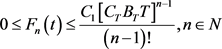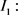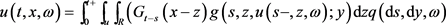(6)(7)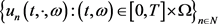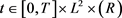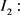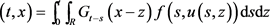(8)(9)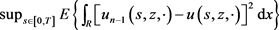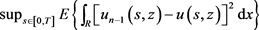是有界的，由收敛控制定理可以得到上述公式(7)和公式(9)的最后一项收敛到0。进而公式(7)和(9)成立。从以上论述可以得到是可以关于t左极右连，且关于x连续。因此，u是方程(1)的解，进而解的存在性得证。

2.4. 唯一性证明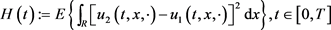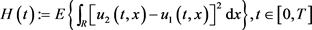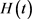关于变量t是左极右连的。因此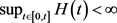，通过解的存在性证明，我们得到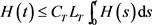。由Gronwall不等式，我们可以得到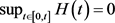，即对于所有的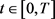和所有的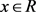得到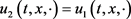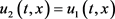，即方程不存在两个不同的解，与原假设矛盾，唯一性得证。

  Knoche, C. (2004) SPDEs in Infinite Dimension with Poisson Noise. Comptesrendus-Mathématique, 339, 647-652. https://doi.org/10.1016/j.crma.2004.09.004  Albeverio, S., Wu, J.L. and Zhang, T.S. (1998) Parabolic SPDEs Driven by Poisson White Noise. Stochastic Processes and Their Applications, 74, 21-36. https://doi.org/10.1016/S0304-4149(97)00112-9  Cardon-Weber, C. (1999) Large Deviations for a Burg-ers’-Type SPDE. Stochastic Processes and Their Applications, 84, 53-70. https://doi.org/10.1016/S0304-4149(99)00047-2  von Renesse, M.-K. and Scheutzow, M. (2010) Existence and Uniqueness of Solutions of Stochastic Functional Differential Equations. Random Operators and Stochastic Equa-tions, 18, 267-284. https://doi.org/10.1515/rose.2010.015  Röckner, M. and Zhang, T. (2011) Stochastic 3D Tamed Navier-Stokes Equations: Existence, Uniqueness and Small Time Large Deviation Principles. Journal of Dif-ferential Equations, 252, 716-744. https://doi.org/10.1016/j.jde.2011.09.030  陶文健. 随机波动方程解的渐近行为[D]: [硕士学位论文]. 湘潭: 湘潭大学, 2020.  朱星亮. 一类半线性随机波动方程解的存在唯一性[D]: [硕士学位论文]. 成都: 西南交通大学, 2012.  成丹. 泊松白噪声驱动的一维随机波动方程[D]: [硕士学位论文]. 合肥: 中国科学技术大学, 2015.